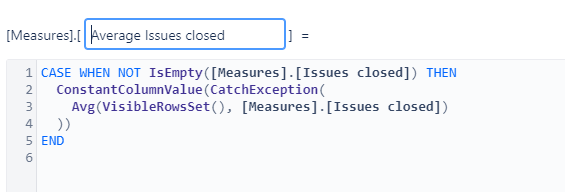# How can I get the Measure of an Average of the row vs the Column total

Here is the Measure currently.I want the report to to show in the columns the number of Closed issues in the month; a Cumulative Sum; then a Daily Average with the Assignees as the Rows.
Gene

Hi,

I imagine the following report:

1. Assignees in rows
2. Time dimension in Pages (select the month you need)
3. in columns, measure “Issues closed” and calculated measure for each assignee’s average daily closed issues within the select month.

The calculation formula for such a measure:

``````[Measures].[Issues closed]
/
DateDiffDays([Time].CurrentHierarchyMember.StartDate,
[Time].CurrentHierarchyMember.NextStartDate)
``````

I am unsure if I get the idea about the cumulative closed issues: what would you need to calculate? The standard cumulative sum (option Add calculated > Cumulative sum) would calculate the cumulative sum of rows (assignees). Do you need that or something else?

How the report could look:

Best,
Ilze, support@eazybi.com